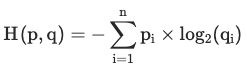## Cross-EntropyCross-entropy measures the average number of bits required to identify an event if you had a coding scheme optimised for one probability distribution $q$, where the true probability distribution was actually $p$.

It's the same as Information Entropy but measuring what happens if you have are identifying messages using a different probability distribution.

Expressed as: $H(p, q)=-\sum\limits_{i=1}^{n} p_{i} \times log_2(q_{i})$

Wikipedia (2021)

#### References

Wikipedia. Cross entropy. Wikipedia, July 2021. URL: https://en.wikipedia.org/wiki/Cross_entropy.